#### 期刊菜单

Finite Element Simulation of Multilayer Functionally Gradient Shaft Wall

Abstract: In this paper, the shaft wall of functionally gradient vertical shaft is divided into multi-layer coaxial cylinders by using the idea of laminating model, and numerical simulation is carried out with the finite element software ABAQUS to analyze the influence of the change of elastic modulus difference between layers on the mechanical properties of multi-layer functionally gradient shaft wall. According to this, we obtain the optimal difference of elastic modulus between layers when the stress distribution inside the cylinder is most uniform, and explore the variation of the optimal difference of elastic modulus between layers when the number of layers of functionally gradient shaft wall is different and the causes of the difference are discussed. The result shows that when the different layers of functionally graded sidewall are corresponding to the most appropriate elastic modulus difference between layers, the function gradient vertical shaft sidewall internal Mises stress reaches relatively uniform, which is effective in relieving the stress concentration phenomenon, the inside of the wall at the same time to minimize the maximum stress of the inside of the wall and the most appropriate elastic modulus difference between layers decreases as the number of layers increases. This study is of great significance to determine the number of dividing layers and the selection of concrete label of each layer of functionally graded shaft wall in practical engineering application.

1. 引言

2. 有限元模型建立Table 1. Calculation model parameters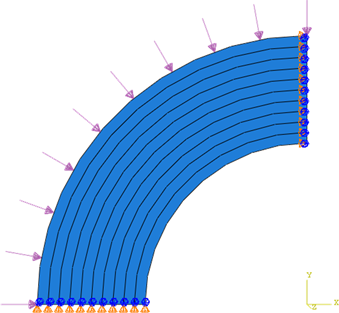Figure 1. Schematic diagram of calculation model when n = 10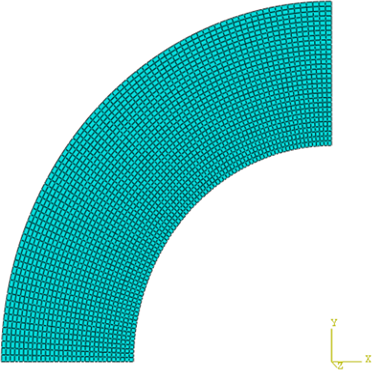Figure 2. Grid division diagram when n = 10

3. 仿真结果分析

3.1. n = 6时Mises应力云图分析(a) 层间弹性模量差值为1 GPa(b) 层间弹性模量差值为10 GPa(c) 层间弹性模量差值为5 GPa(d) 层间弹性模量差值为6 GPa

Figure 3. Mises stress cloud at n = 6Table 2. Maximum stress and minimum stress calculated with the change of elastic modulus difference between layers when n = 6

3.2. n = 10时Mises应力云图分析(a) 层间弹性模量差值为1 GPa(b) 层间弹性模量差值为10 GPa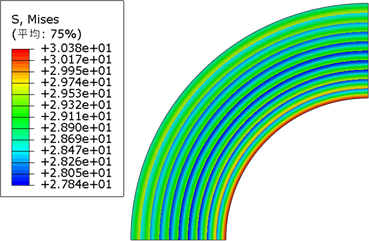(c) 层间弹性模量差值为3 GPa(d) 层间弹性模量差值为4 GPa

Figure 4. Mises stress cloud at n = 10Table 3. Maximum stress and minimum stress calculated with the change of elastic modulus difference between layers when n = 6

3.3. n = 15时Mises应力云图分析(a) 层间弹性模量差值为1 GPa(b) 层间弹性模量差值为10 GPa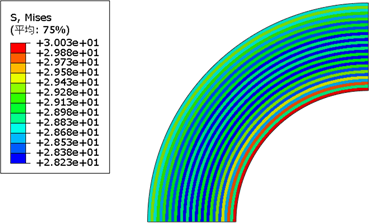(c)层间弹性模量差值为2 GPa(d) 层间弹性模量差值为3 GPa

Figure 5. Mises stress cloud at n = 15Table 4. Maximum stress and minimum stress calculated with the change of elastic modulus difference between layers when n = 15

4. 仿真结果原因分析Table 5. Changes of Mises stress, radial stress and circumferential stress calculated with the difference of elastic modulus between layers at n = 10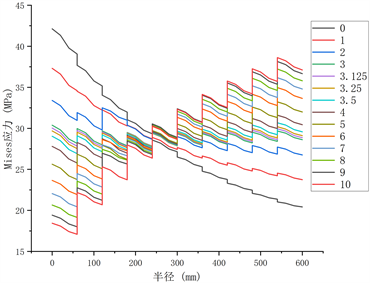(a) Mises应力与圆筒半径关系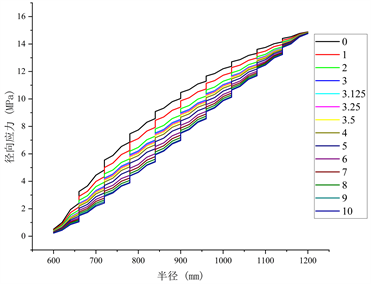(b) 径向应力与圆筒半径关系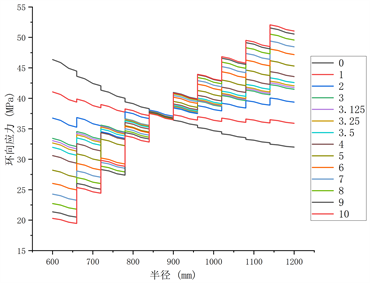(c) 环向应力与圆筒半径关系

Figure 6. Relationship between Mises stress, radial stress, annular stress and cylinder radius at n = 10

5. 结论

1、各种层数均对应一个最合适的层间弹性模量差值，使得功能梯度立井井壁Mises应力达到相对均匀(即有效缓解了井壁内侧的应力集中现象)，井壁内部的最大应力达到最小。

2、最合适层间弹性模量差值随着层数增加而减小，即层数15的最适层间弹性模量差值 < 层数10的最适层间弹性模量差值 < 层数6的最适层间弹性模量差值。

3、井壁内部各点环向应力均大于径向应力，并且随着层间弹性模量差值的增大，各点环向应力的变化十分显著，但径向应力变化很小，即环向应力占据影响Mises应力的主导地位，决定了两者合成的Mises应力的变化趋势。即环向应力随着层间弹性模量差值的增大，从初的应力内侧大外侧小，到相对均匀，再到内侧小外侧大，从而引起环向应力和径向应力合成的Mises应力发生与环向应力相同的变化趋势。

4、在实际工程运用中，可利用上述得出的各分层数下最合适层间弹性模量差值来设计功能梯度立井井壁，确定各层混凝土的弹性模量，从而配置符合条件的混凝土。并且设计的井壁分层数越多，需要配置的混凝土的弹性模量整体上也就越低，可以在达到缓解井壁内侧应力集中的同时达到降低成本的目的，但井壁的分层数也不应过多，否则会导致各层厚度过小，不便于施工中浇筑混凝土。

  姚直书, 程桦, 荣传新. 深冻结井筒内层钢板高强钢筋混凝土复合井壁试验研究[J]. 岩石力学与工程学报, 2008, 27(1): 158-165.  Zhang, N., Lu, A., Li, C.C., et al. (2017) Support Performance of Functionally Graded Concrete Lining. Construction and Building Materials, 147, 35-47. https://doi.org/10.1016/j.conbuildmat.2017.04.161  Koizumi, M. (1997) FGM Activities in Japan. Composites Part B: Engineering, 28, 1-4. https://doi.org/10.1016/S1359-8368(96)00016-9  Shi, Z., Zhang, T. and Xiang, H. (2007) Exact Solutions of Heterogeneous Elastic Hollow Cylinders. Composite Structures, 79, 140-147. https://doi.org/10.1016/j.compstruct.2005.11.058  Xiang, H.J., Shi, Z.F. and Zhang, T.T. (2006) Elastic Analyses of Heterogeneous Hollow Cylinders. Mechanics Research Communications, 33, 681-691. https://doi.org/10.1016/j.mechrescom.2006.01.005  Yang, Y.Y. (2000) Time-Dependent Stress Analysis in Functionally Graded Materials. International Journal of Solids and Structures, 37, 7593-7608. https://doi.org/10.1016/S0020-7683(99)00310-8  Horgan, C.O. and Chan, A.M. (1999) The Pressurized Hollow Cylinder or Disk Problem for Functionally Graded Isotropic Linearly Elastic Materials. Jounal of Elasticity, 55, 43-59. https://doi.org/10.1023/A:1007625401963  张宁, 张雨辰, 吕爱钟, 陈旭光. 一种功能梯度混凝土复合井壁及制作方法[P]. 中国专利, CN111287754A. 2020-06-16.  张宁. 功能梯度混凝土立井井壁承载性能研究[D]: [博士学位论文]. 北京: 华北电力大学, 2012.  许冲. 功能梯度立井井壁力学性能及其设计理论研究[D]: [硕士学位论文]. 徐州: 中国矿业大学, 2021.  李德春, 许冲, 崔振东, 张通通, 杨杰, 宋刚, 杨金宏, 陈金明, 徐香庆, 张照伟. 一种多层功能梯度立井井壁结构及施工工艺[P]. 中国专利, CN112031777A. 2020-12-04.  李德春, 张通通, 崔振东, 等. 弹性模量环向梯度变化的功能梯度井壁结构及施工方法[P]. 中国专利, CN112360467A. 2021-02-12.  吕爱钟, 张路青. 用于提高立井井壁弹性极限承载力的梯度功能材料反演[C]//中国岩石力学与工程学会. 第十届全国岩石力学与工程学术大会论文集: 2008年卷. 北京: 中国电力出版社, 2008: 138-144.